#### Test of Reasoning - Relations

##### Click on the option you think is right and then check by clicking on the Show Answer button. You can change your option as many times as you like.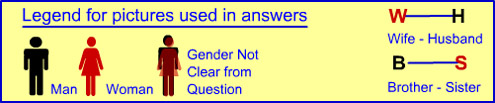### Score 0

1. A is B’s brother. C is D’s father. E is B’s mother. A and D are brothers. How is E related to C?

Answer (d).2. B is the brother of A, whose only sister C is mother of D. E is maternal grandmother of D. How is B related to E?

Answer (c).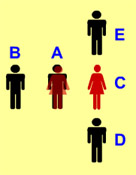3. Raju is the brother of Alok. Sunita is the sister of Sunil. Alok is the son of Sunita. How is Raju related to Sunil?

Answer (a).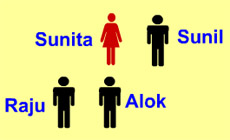4. A and B are sisters, R and S are brothers. A’s daughter is R’s sister. What is B’s relation to S?

Answer (d).5. E is the sister of B, A is the father of C, B is the son of C. How is A related to E?

Answer (a).6. A is B’s brother, C is A’s mother, D is C’s father, E is B’s son. How is D related to E?

Answer (d).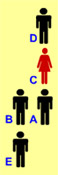7. B is D’s mother and C is D’s brother. H is E's daughter whose wife is D. How are E and C related?

Answer (b).8. Given that A is the mother of B. C is the son of A. D is the brother of E. E is the daughter of B. Who is the grandmother of D?

Answer (a).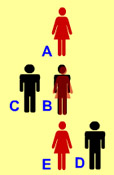9. A is D’s brother. D is B’s father. B and C are sisters. How is A related to C?

Answer (d).10. Savita is the niece of Ashok, whose is mother is Lavanya. Lavanya's mother is Kavita. Kavita's husband is Govardhan. Padma is the mother-in-law of Govardhan. How is Savita related to Govardhan?

Answer (c).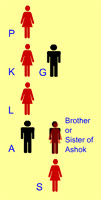11. A is B’s sister. C is B’s mother, D is C’s father, E is D’s mother, then how A is related to D?

Answer (b).12. If B is the brother of A, D is the daughter of A, L is the sister of B, M is the brother of D, Who is the uncle of M?

Answer (d).13. Reena is the daughter-in-law of Vinay and sister-in-law of Suresh. Manoj is the son of Vinay and only brother of Suresh. Find the relation between Reena and Manoj.

Answer (a).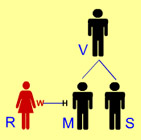14. Suresh introduces a man as “He is the son of the woman who is the mother of the husband of my mother”. How is Suresh related to the man?

Answer (d).15. B is A's brother. C is A's father. D is C's sister and E is D's mother. How is B related to E?

Answer (a).